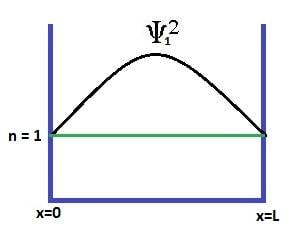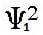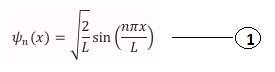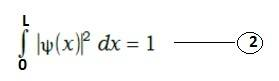# Charge Density in Quantum well Sub-bands.

• kpsr

## Homework Statement

I am trying to calculate the charge density in the first subband (n=1) of the quantum well of length L as shown in the below figure.hereis the electron wave function for the first sub-band and its value (from relevant equation 1) is given as
Ψ12(x) = 2/L*sin2(πx/L)

from Schrodinger equation total electron charge density is given by
n(x) = N1×Ψ12(x)
where N1 is the electron charge density in the first subband(n=1).

hence for charge density at x = L/2 the above equation becomes
n(L/2) = N1×Ψ12(L/2) = N1×2/L*sin2(π/2) --result 1

But from wave function normalization,that is the probability of finding electron between 0 and L is one(from relevant equation 2)
Ψ12(L/2) ≈1, then the total electron charge density at x=L/2 is n(L/2) = N1×1 --result 2

2. Homework Equations

wave-function for the electrons in the quantum well sub bandswave function normalization(that is the probability of finding electron between 0 and L is one)## The Attempt at a Solution

When i made attempts for the solutions i got two results result 1 and result 2 as above.
Now my question is which is the correct result from these two..?

hereis the electron wave function for the first sub-band and its value (from relevant equation 1) is given as
Ψ12(x) = 2/L*sin2(πx/L)

from Schrodinger equation total electron charge density is given by
n(x) = N1×Ψ12(x)
where N1 is the electron charge density in the first subband(n=1).

I think the the wave function provide you the info about the probability of finding the charge(electron) in the one dimensional well in a certain state- so the density can be calculated by multiplying the charge with modulus square of the wave function between a point x and x+dx =that should be density at x- naturally as you show the nature of wave function its modulus square will be maximum at x= L/2 but the charge will not be always found at this point - as the total probability should be unity for a normalized wave function .
and at other points between 0 and L there exists finite probability density.
so you can not have two answers at a point-density should / will be unique.